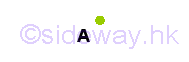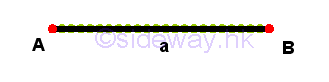output.to from Sideway
Geometry

Euclid's Elements

Geometric Element

Draft for Information Only

# Content

`  The Fundamentals of Geometric Figures    Dimensions     Elements of Geometric Figures`

## The Fundamentals of Geometric Figures

Geometry is the study of figures. There are many ways to describe a figure

### Dimensions

In general, a dimension is a phsical measurement of size, length, or distance in one direction. A dimension is also a descriptive measurement of the intrinsic property of an object.

### Elements of Geometric Figures

The fundamental notions of geometry.

1. A point is a primitive notion that has no dimensional attribute, 0-dimensional. A point can lie in a line, in a line, or in space.2. A line or straight line is a length notion that has one linear dimensional attribute called length, 1-dimensional. A line has no breadth, and thickness and can lie in a plane or in space. The extremities of a line are points.3. A plane, flat plane or Euclidean plane is an area notion that has two linear dimensional attributes called length and breadth, 2-dimensional. A plane has no thickness and can lie in space. The extremities of a plane are lines and the extremities of a line are points. Therse points are called vertices.4. A space, cuboid space or Euclidean space is an volume notion that has three linear dimensional attributes called length, breadth, and thickness, 3-dimensional. The extremities of a plane are lines and the extremities of a line are points. The extremities of a space are planes, the extremities of a plane are lines and the extremities of a line are points. Therse points are called vertices.

The fundamental objects of geometry for describing geometric figures.

1. A vertice is a 0-dimensional object but a vertice can lie in higher dimensional. A vertice itself has no length, breadth, and thickness.

2. A curve is a 1-dimensional object but a curve can lie in higher dimensional. A curve itself has no breadth and thickness.

3. A surface is a 2-dimensional object but a surface can lie in higher dimensional. A surface itself has no thickness.

4. A solid is a 3-dimensional object and a solid can lie in space only.

The fundamental elements of geometric figures.

1. A two dimensional figure, also called plane figure or planar figure, is a 2-dimensional object lying in a single plane but a planar figure can lie in higher dimensional.

A planar figure is a set of line segments and curve segments, which are also called sides and arcs. The sides and arcs of a two dimensional figure are called the edges of the figure. The edges themselves are one-dimensional object, but the edges lie in the two-dimensional plane forming a two dimensional figure. The interections of the edges are called the vertices or corners. Usually a vertex is refered to the end point of at least two edges, sometime a vertex can also be used to name the end point of the loose end of an edge. These vertices or points are zero-dimensional, but points lie in a one dimensional line in the two-dimensional plane.

2. A three dimensuon figure, also called solid figure, is a 3-dimensional object lying in three dmensional space only.

A solid figure is a set of plane regions and surface regions, which are also called surfaces. The surfaces of a three dimensional figure are called the faces of the figure. The face themselves are two-dimensional object, but the faces lie in the three dimensional space forming a three dimensional figure. The sides and arcs that are the edges of the faces of the solid figure are called the edges of the figure. The edges themselves are one-dimensional object, but the edges lie in the two-dimensional space forming a two dimensional figure in three dimensional space. Similarly, the interections of the edges are called the vertices or corners of the solid figure. These vertices are zero-dimensional, but points lie in the two-dimensional plane in three dimensional space.

ID: 150200007 Last Updated: 10/2/2015 Revision: 0 Ref:References

1. Hilbert, D. (translated by Townsend E.J.), 1902
2. Moore, E.H., 1902
3. Fitzpatrick R. (translated), Heiberg J.L. (Greek Text), Euclid (Author), 2008Home 5

Management

HBR 3

Information

Recreation

Culture

Chinese 1097

English 337

Computer

Hardware 149

Software

Application 187

Numeric 19

Programming

Web 757

CSS 1

HTML

Knowledge Base

OS 389

MS Windows

Knowledge

Mathematics

Algebra 20

Geometry 18

Calculus 67

Engineering

Mechanical

Rigid Bodies

Statics 92

Dynamics 37

Control

Physics

Electric 10